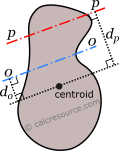## Parallel axes Moments of Inertia

This tool calculates the moment of inertia Ip (second moment of area) of a planar shape, about an axis p-p , given the moment of inertia of the same shape relative to an axis 0-0, parallel to the first one. Enter area of the shape A, the moment of inertia I0 about axis 0, and the distances d0, dp of the two axes from centroid, as shown in figure. The calculated results will have the same units as your input. Please use consistent units for any input.

 A = I0 = d0 = dp = The result is: Ip =ADVERTISEMENT

## Definitions

### Parallel Axes Theorem

The moment of inertia of any shape, in respect to an arbitrary, non centroidal axis, can be found if its moment of inertia in respect to a centroidal axis, parallel to the first one, is known. The so-called Parallel Axes Theorem is given by the following equation:

where I' is the moment of inertia in respect to an arbitrary axis, I the moment of inertia in respect to a centroidal axis, parallel to the first one, d the distance between the two parallel axes and A the area of the shape. Applying the above formula, for two parallel axes, at distances d0 and dp from centroid, the following relation between the moments of inertia I0 and Ip relative to each axis respectively, is found:

### Dimensions

The dimensions of moment of inertia (second moment of area) are .

### Mass moment of inertia

In Physics the term moment of inertia has a different meaning. It is related with the mass distribution of an object (or multiple objects) about an axis. This is different from the definition usually given in Engineering disciplines (also in this page) as a property of the area of a shape, commonly a cross-section, about the axis. The term second moment of area seems more accurate in this regard.

### Applications

The moment of inertia (second moment or area) is used in beam theory to describe the rigidity of a beam against flexure (see beam bending theory). The bending moment M applied to a cross-section is related with its moment of inertia with the following equation:

where E is the Young's modulus, a property of the material, and κ the curvature of the beam due to the applied load. Beam curvature κ describes the extent of flexure in the beam and can be expressed in terms of beam deflection w(x) along longitudinal beam axis x, as: . Therefore, it can be seen from the former equation, that when a certain bending moment M is applied to a beam cross-section, the developed curvature is reversely proportional to the moment of inertia I. Integrating curvatures over beam length, the deflection, at some point along x-axis, should also be reversely proportional to I.